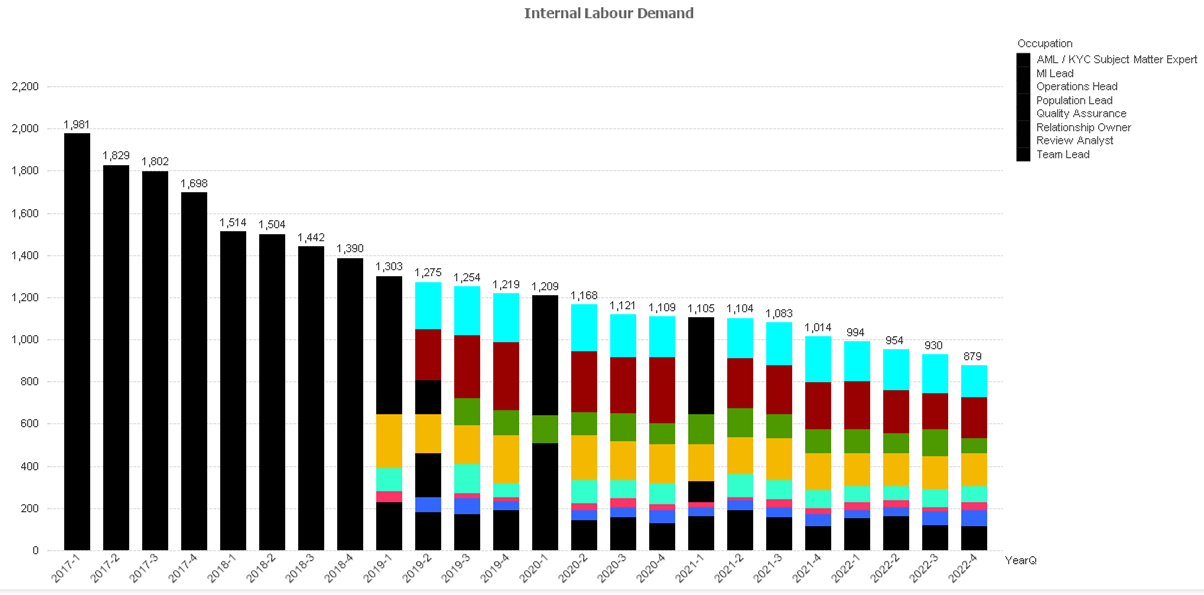# QlikView App Dev

Discussion Board for collaboration related to QlikView App Development.

Announcements
QlikWorld 2023, a live, in-person thrill ride. Save \$300 before February 6: REGISTER NOW!
cancel
Showing results for
Did you mean:Not applicable

## Stacked bar chart colour query

Hi All,

I have a dimension (Occupation) that appears on 3 or 4 separate charts throughout my dashboard. I have set up a variable called vColourOccupation which has the following if statement:

if(Occupation='Quality Assurance',RGB(245,184,0),
if(Occupation='Relationship Owner',RGB(77,153,0),
if(Occupation='Review Analyst',RGB(153,0,0),
RGB(0,0,0))))))))

In the background colour piece of the expression I have put the variable in here. However when I do this and open my dashboard it looks like the following:Whenever I select one of these bars and unselect it then it fixes this issue and looks like the following:Anyone any ideas why this would be? The expression is the following:

//Baseline

sum({<YearQ={'2017-1','2017-2','2017-3','2017-4'}, Attrition=, Retirement=>}[Demand C])

+
sum({<Year={'2018'}, Attrition=, Retirement=>}[Demand C])*vMixD2014
+
sum({<Year={'2019'}, Attrition=, Retirement=>}[Demand C])*vMixD2015
+
sum({<Year={'2020'}, Attrition=, Retirement=>}[Demand C])*vMixD2016
+
sum({<Year={'2021'}, Attrition=, Retirement=>}[Demand C])*vMixD2017
+
sum({<Year={'2022'}, Attrition=, Retirement=>}[Demand C])*vMixD2018

+
sum({<Year={'2018'}, Attrition=, Retirement=>}[Demand F])*(1-vMixD2014)
+
sum({<Year={'2019'}, Attrition=, Retirement=>}[Demand F])*(1-vMixD2015)
+
sum({<Year={'2020'}, Attrition=, Retirement=>}[Demand F])*(1-vMixD2016)
+
sum({<Year={'2021'}, Attrition=, Retirement=>}[Demand F])*(1-vMixD2017)
+
sum({<Year={'2022'}, Attrition=, Retirement=>}[Demand F])*(1-vMixD2018)

//Variables

+
sum({<Year={'2017'}, Attrition=, Retirement=>}[Demand C])*vMixD2014*(vNewClients - vAutomation + vRisk + vRegulation)
+
sum({<Year={'2018'}, Attrition=, Retirement=>}[Demand C])*vMixD2014*(vNewClients - vAutomation + vRisk + vRegulation)
+
sum({<Year={'2019'}, Attrition=, Retirement=>}[Demand C])*vMixD2015*(vNewClients - vAutomation + vRisk + vRegulation)
+
sum({<Year={'2020'}, Attrition=, Retirement=>}[Demand C])*vMixD2016*(vNewClients - vAutomation + vRisk + vRegulation)
+
sum({<Year={'2021'}, Attrition=, Retirement=>}[Demand C])*vMixD2017*(vNewClients - vAutomation + vRisk + vRegulation)
+
sum({<Year={'2022'}, Attrition=, Retirement=>}[Demand C])*vMixD2018*(vNewClients - vAutomation + vRisk + vRegulation)

+
sum({<Year={'2017'}, Attrition=, Retirement=>}[Demand F])*(1-vMixD2014)*(vNewClients - vAutomation + vRisk + vRegulation)
+
sum({<Year={'2018'}, Attrition=, Retirement=>}[Demand F])*(1-vMixD2014)*(vNewClients - vAutomation + vRisk + vRegulation)
+
sum({<Year={'2019'}, Attrition=, Retirement=>}[Demand F])*(1-vMixD2015)*(vNewClients - vAutomation + vRisk + vRegulation)
+
sum({<Year={'2020'}, Attrition=, Retirement=>}[Demand F])*(1-vMixD2016)*(vNewClients - vAutomation + vRisk + vRegulation)
+
sum({<Year={'2021'}, Attrition=, Retirement=>}[Demand F])*(1-vMixD2017)*(vNewClients - vAutomation + vRisk + vRegulation)
+
sum({<Year={'2022'}, Attrition=, Retirement=>}[Demand F])*(1-vMixD2018)*(vNewClients - vAutomation + vRisk + vRegulation)

Would anything here be affecting it?

1 Solution

Accepted SolutionsMVP

Try replacing Occupation with Only({1}Occupation) in your variable

talk is cheap, supply exceeds demand
4 RepliesMVP

Try replacing Occupation with Only({1}Occupation) in your variable

talk is cheap, supply exceeds demandNot applicable
Author

Hi Gysbert,

This worked perfectly, could you advise on what that is actually doing out of curiosity?

ThanksMVP

If you select one Occupation value then the if statement will only encounter that selected value. So you get only a color for the selected value. By explicitly using an aggregation function like Only() it's possible to add a set condition to overrule the user selection. The {1} means that all user selections should be ignored.

talk is cheap, supply exceeds demandNot applicable
Author

Hi Gysbert Wassenaar,

Really appreciate that, a bit new to all this so the more knowledge I can get the better.

Thanks again

DavidCommunity Browser